# Differentiating Functions with Sage!

## Primary tabs

This is the third lesson in five where we are discussing Sage and how it can be used to make your Calculus life easier. In the first lesson you learned how to create your own account and how to create a worksheet. In the second lesson you learned how to plot functions with Sage. In this lesson we are going to learn how to calculate derivatives. So to get started log into your account, create a new worksheet and label it derivatives. You can do this by clicking the "New" button, typing "derivatives" in the text box and hitting the "Sage Worksheet" button just as we did in the first lesson.

Now you should have a worksheet where we can type in commands. Let's get started with finding the derivative of the polynomial $f(x)=ax^2+bx+c$. To do this just type

var("x a b c")

and
f=a*x^2+b*x+c

into the worksheet. The first line defines variables. Don't worry too much about this. Just trust us that it needs to be done. Now we are going to introduce a new command. Type "show(f)" on the next line. This will give you a pretty representation of the function as you would write it on paper. Now hit the run button or shift+return to see the results. You should have something like this: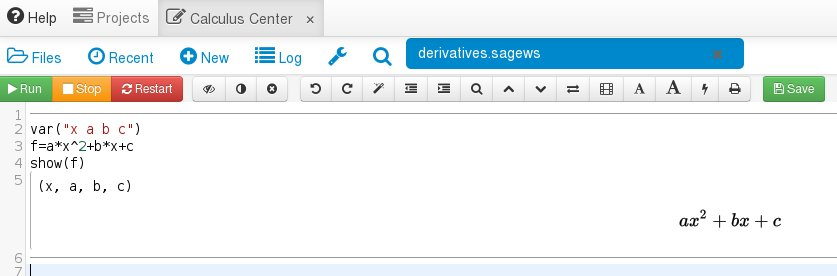Now let's differentiate $f(x)$. After show(f) type "dfdx=f.diff()" with "show(dfdx)" below it. Now hit the run button or shift+return to execute. You should get something like this: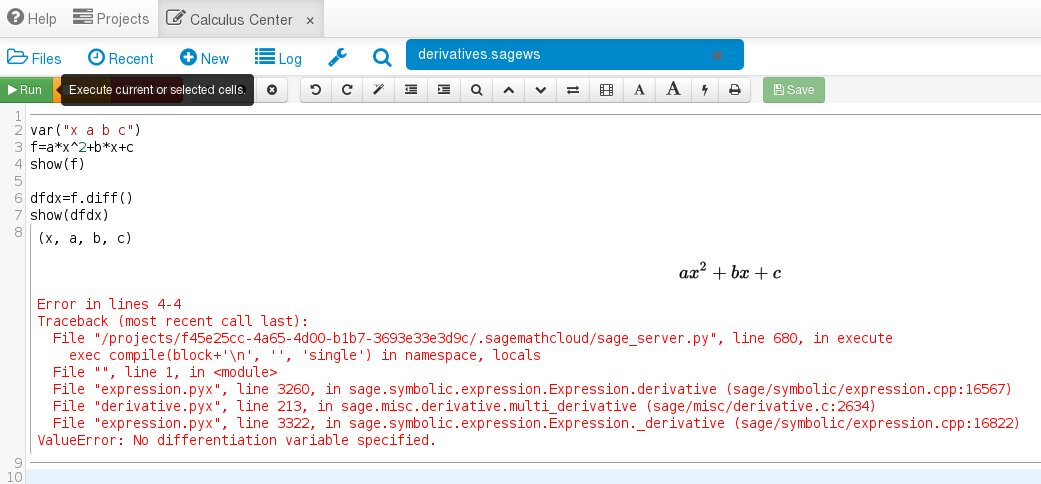Notice the warning at the bottom which says "ValueError: No differentiation variable specified." Because we have four variables it did not know which one to differentiate the function with. We had $x$ in our heads, but did not specify that. So let's do it properly by typing "f.diff(x)" instead of "f.diff()". Now you should see the correct answer which is $2ax+b$. Here is what you should see in Sage: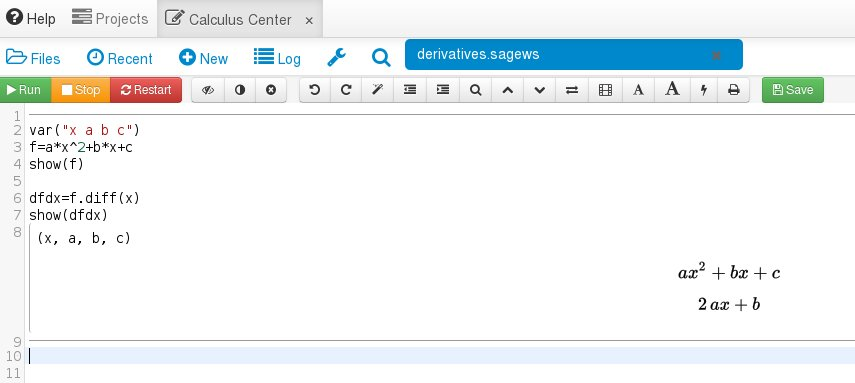And of course we can find the second derivative by another use of diff(x):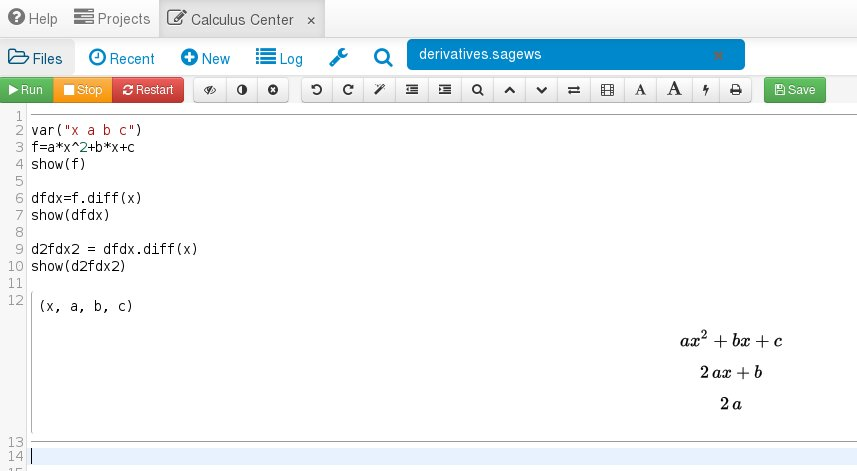This has all been symbolic which is great, but what if we have actual numbers instead of the variables $a$, $b$, and $c$? Well that is very easy. Let's say $a=3$, $b=-2$ and $c=1$. This is how you do it: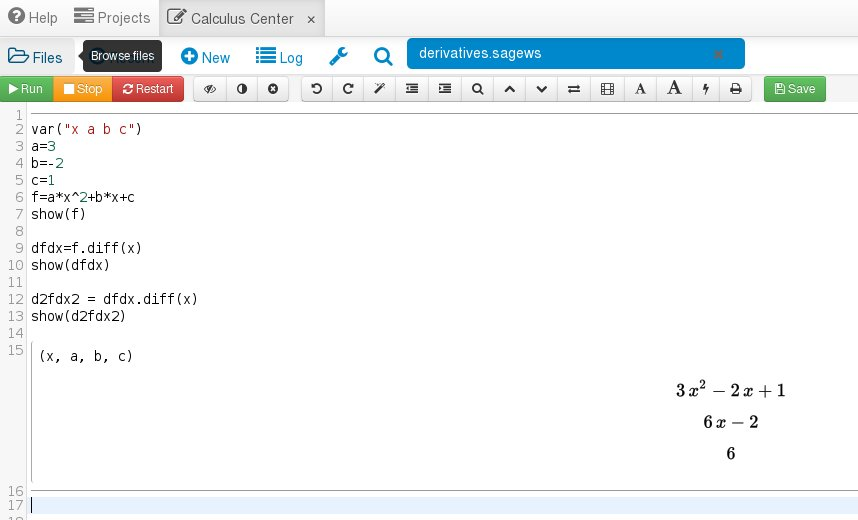We can plot the function and its derivatives: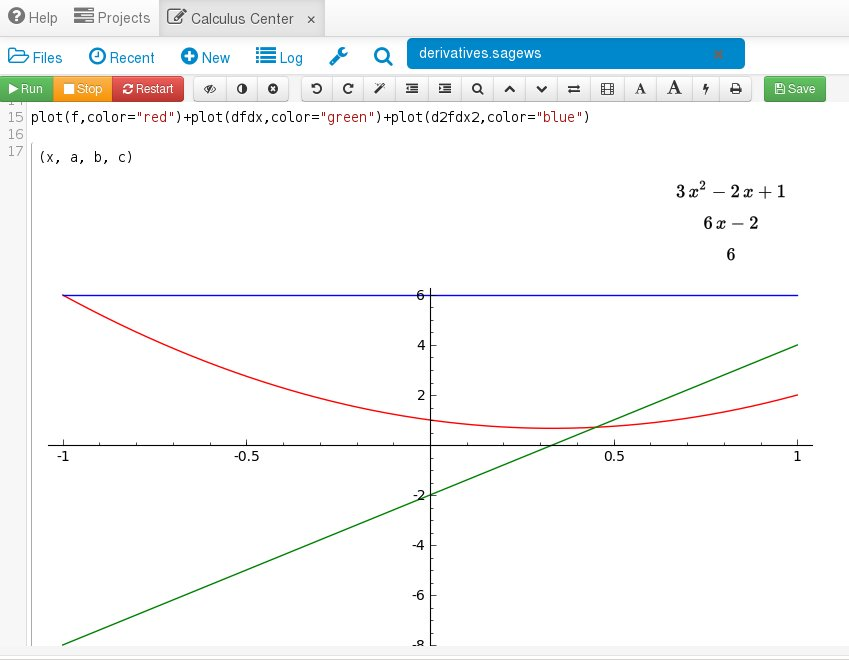Finally, let's plot the function and its tangent line: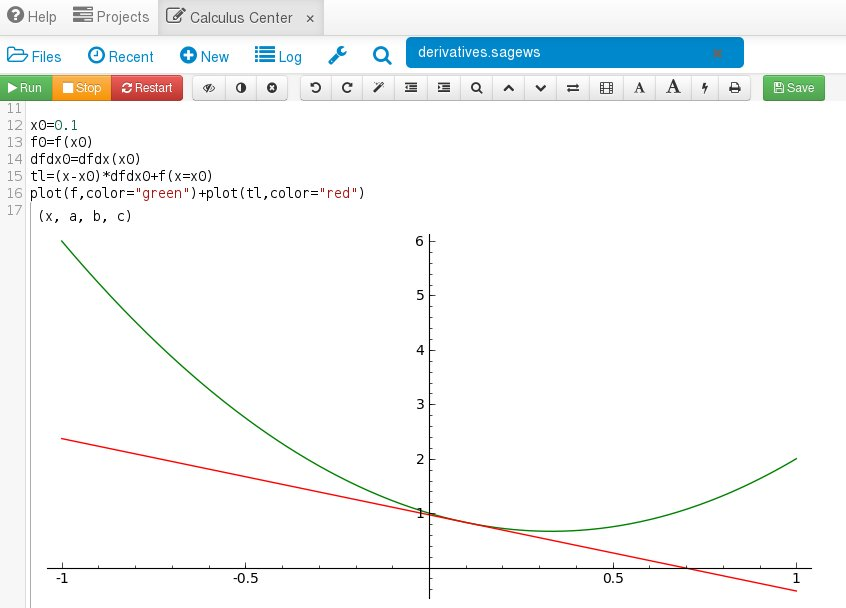Tomorrow we will look at how to integrate functions with Sage. Finally, if you want to jump to the other blog posts for Sage Week then here they are: